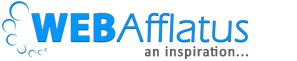Aside

# Introduction to R Package Opera – A very powerful ensemble packageFew years back, I was working on a Demand forecasting project where I was dealing with thousands of combinations of Brand-SKU-Store combination. The challenge was to improve existing accuracy to provide better forecasts of each SKU at a store level. Even if you are dealing with 100 SKUs and 200 stores there will be 20,000 combinations and you can’t selectively pick a model for each combination. We tried various individual techniques such as ARIMA, ETS, UCM, NN, and many more but couldn’t meet the accuracy benchmark. Then one of my seniors introduced me to the OPERA package and asked me to research and optimize it for our needs. I successfully implemented it and today I will talk about the same package with detailed R code.

What is an ensemble technique?

In nutshell, ensemble techniques combine multiple individual techniques to build a more powerful technique, which allows us to make a better prediction and improve results compared to a single model. In ML courses, we might have read about bagging and boosting where many decision trees are combined to build powerful Random forest and XGb models.

In the above examples, you are combining similar kinds of models, like we are combining multiple decision trees to build a stronger model. But what if we want to combine different kinds of models like UCM, GAM, NN, Random forest, etc. One way will be to give weight to each of them, but it will be less flexibility.
To overcome these gaps, we are introducing the OPERA package which might be useful in many scenarios.

OPERA TheoryOPERA – How does it work?Important termologies:

1. Experts: Set of individual technique
2. Mixture: to build the algorithm object
3. Predict: to make the prediction by using the algorithm
4. Oracle: to evaluate the performance of the experts and compare the performance of the combining algorithm

Mixture function():

```mixture(
Y = NULL,
experts = NULL,
model = "MLpol",
loss.type = "square",
coefficients = "Uniform",
awake = NULL,
parameters = list()
)
```

Important Hyperparameters:
• Y: data stream to predict
• Experts: the set of experts
• Model: aggregation methods (EWA, FS, Ridge, MLpol, OGD)
• Loss.type: the loss function (absolute, square, percentage,…)

Oracle function():

```oracle(
Y,
experts,
model = "convex",
loss.type = "square",
awake = NULL,
lambda = NULL,
niter = NULL,
...
)
```

Important Hyperparameters:
• ‘expert’: The best fixed (constant over time) expert oracle.
• ‘convex’: The best fixed convex combination (vector of non-negative weights that sum to 1)
• ‘linear’: The best fixed linear combination of expert
• ‘shifting’: It computes for all number \$m\$ of switches the sequence of experts with at most \$m\$ shifts that would have performed the best to predict the sequence of observations in Y.

In the below image, you can see how important to experts varies across time frame.OPERA Implementation using Real Data

```## Using Tourist data
dt <- ts(dt\$United.Kingdom, start = c(1998,4), frequency = 4)

train <- window(dt, end = c(2009,4), start = c(1999,1))
test <- window(dt,start = c(2010,1))

forecast.period <- length(test)
```

You can use the attached R code for detail code. Here I am giving a glance to important steps. We are using Tourist data which you can download from here. Download data

```########### Example 2: COMPLETE MODEL - TRAINING WITH 3 EXPERTS

expert.1 <- forecast(auto.arima(train), h = forecast.period)
expert.2 <- forecast(ets(train), h = forecast.period)
expert.3 <- forecast(tbats(train), forecast.period)
```
```########### Building Oracle funciton
MLpol <- mixture(Y = train, experts = train.experts,
loss.type = "square", model = "MLpol")
oracle.convex <- oracle(Y = train, experts = train.experts,
loss.type = "square", model = "convex")
```
```########### Prediciting using above trained MLpol function
z <- ts(predict(MLpol, test.experts, y = null,
online = F, type = "response"), start = c(2010,1),
frequency = 4)

```
```########### Calculating MAPE
MAPE <- abs(sum(qc.data\$z) - sum(qc.data\$test))/sum(qc.data\$test)
MAPE

```

Limitation and drawbacks

1. As this is an ensemble technique, finding feature importance will be challenging
2. The model tends to overfit on training data and might not perform well on testing data
3. The model weights are static in sequential order and might not work in the same order in testing data

##### Signature

Deepesh Singh## 5 thoughts on “Introduction to R Package Opera – A very powerful ensemble package”

1.360DigiTMGIN

Great Article it its really informative and innovative keep us posted with new updates. its was really valuable. thanks a lot.

2.Nina Kotze

HI Deepesh,
Looking forward to follow you & your career!
Nina

•Deepesh Singh

Hi Nina – thanks a lot for finding out time and reading my blog. That’s so nice of you. Looking forward to learn many things.
Deepesh Singh

3.elzinkos

Sorry if you find my comment a bit blunt but you miss the whole story of the algorithm : indeed this is an ensemble learning algorithm but its goal is to predict as well as the best convex or linear combination in hindsight, without knowing the data in hindsight (that would be cheating !). For example you can perform online linear regression (with the method “Ridge”) and get results close to (and sometimes even better than) the linear regression you could have performed if you knew all the data in hindsight. On top of that, the theoretical roots of the algorithms are rock solid. Here are some valuable properties that make this family of algorithm outstanding.
If you want an introduction to the subject, here is a presentation Gilles Stoltz, Pierre Gaillard’s PhD advisor : https://www.youtube.com/watch?v=JcEU8lLyUcA
By the way, Pierre Gaillard is now a researcher at the INRIA and the author of the opera package and giving him some credit is the least you could have done.
PS : I’m not Pierre Gaillard but a huge fan of his work.

•Deepesh Singh

Thanks a lot for coming across my blog and sharing your thoughts. What a wonder explanation. Thanks a lot man!